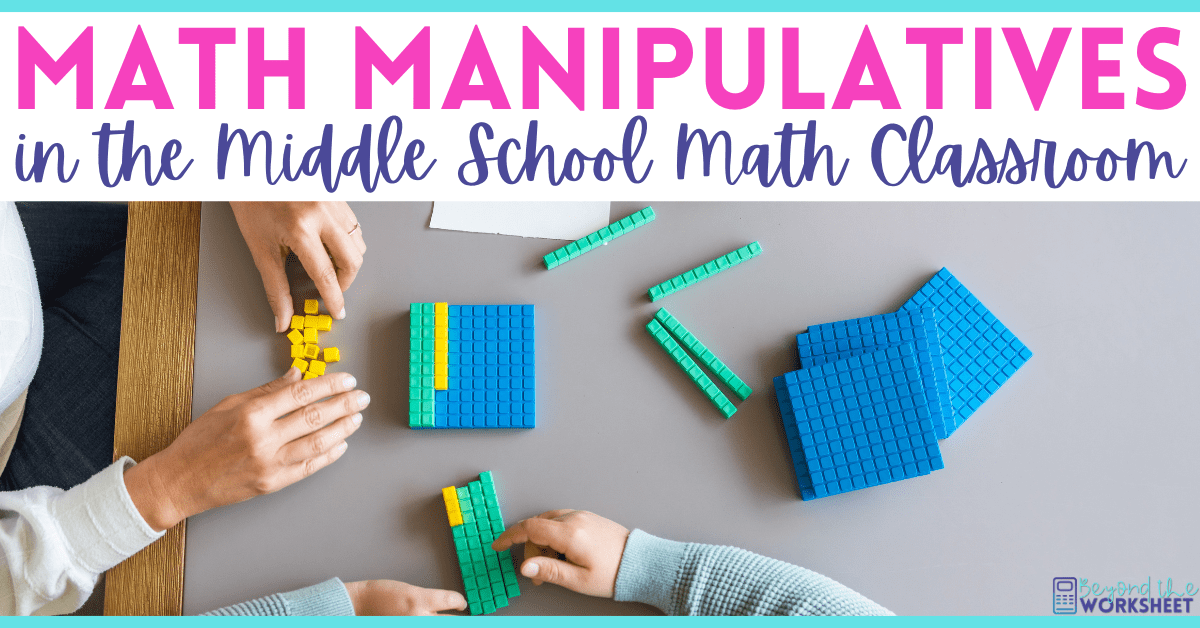Effectively Using Math Manipulatives in Middle School

# Effectively Using Math Manipulatives in Middle SchoolUsing manipulatives in the middle school math classroom is an incredibly effective way to help students understand mathematical concepts. Manipulatives are physical objects that students can move around and interact with in order to visualize and explore mathematical ideas. In this blog post, I will share with you some effective strategies for using manipulatives in the middle school math classroom.

1. Choose the right manipulatives: It is important to choose manipulatives that are appropriate for the grade level and mathematical concepts being taught. For example, base-ten blocks are great for teaching place value and operations with whole numbers, while fraction tiles are useful for teaching fractions and decimals. It is also important to have enough manipulatives for every student to use, so consider purchasing sets or making your own.

2. Introduce the manipulatives: Before using manipulatives in a lesson, it is important to introduce them to the students and explain how they work. Demonstrate how to use them and give students time to explore and play with them. This will help students understand how the manipulatives relate to the mathematical concepts they are learning.

3. Encourage exploration: Manipulatives are meant to be explored and played with. Encourage students to experiment with the manipulatives and come up with their own ideas and strategies for solving problems. This will help them develop a deeper understanding of the mathematical concepts.

4. Use manipulatives to model problems: Manipulatives are a great tool for modeling mathematical problems. For example, if you are teaching multiplication with fractions, you could use fraction tiles to model the problem and show students how to multiply fractions. This will help students visualize the problem and understand how to solve it.

5. Use manipulatives for group work: Manipulatives are great for group work because they encourage collaboration and communication. Assign groups of students to work on a problem together using manipulatives. This will help students develop teamwork skills and learn from one another.

6. Use manipulatives for assessment: Manipulatives can be used for assessment as well. Give students a problem to solve using manipulatives and have them explain their thinking and strategy. This will give you insight into their understanding of the mathematical concepts.

By choosing the right manipulatives, introducing them to students, encouraging exploration, using them to model problems, incorporating them into group work, and using them for assessment, you can help students develop a deeper understanding of mathematical concepts.### Exclusive FREE Math Resources!

Provide your info to instantly receive access to a free resource library and stay up to date with tips, products and insider information!

## Lindsay## Exclusive Freebie

If you’d like a free spin and solve game, click the button above.